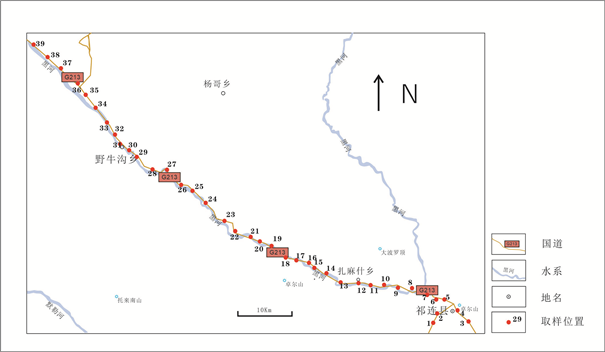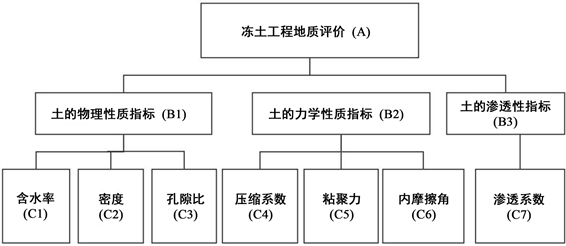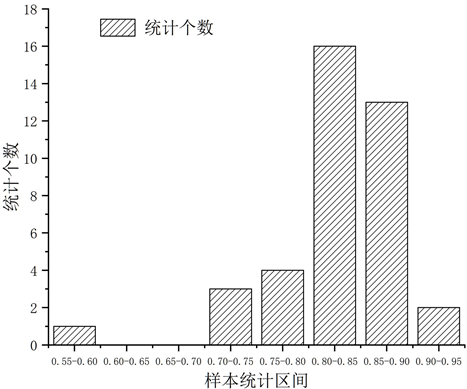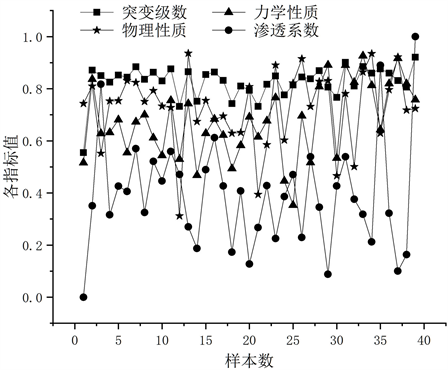#### 期刊菜单

Application of Catastrophe Progression Method in the Stability Evaluation of Subgrade in Permafrost Area—A Case Study of G213 National Highway in Qilian, Qinghai Province
DOI: 10.12677/HJCE.2022.116090, PDF, HTML, XML, 下载: 94  浏览: 125  科研立项经费支持

Abstract: The stability of highway subgrade in permafrost areas has always been a hot topic of theoretical and practical research. In this paper, the catastrophe progression method is proposed to evaluate the subgrade stability of the G213 National Highway in Qilian, Qinghai Province. Based on 39 sets of geotechnical test data, starting from 7 bottom control indexes such as cohesion, internal friction angle, compressibility, natural density, void ratio, water content, and permeability coefficient, we comprehensively consider the impact of each control index on the geotechnical properties of geotechnical engineering. The results show that the catastrophe progression method can represent the engineering property level. The mechanical properties, physical properties, and permeability coefficient of soil mass are sensitive to different grades of engineering properties of soil mass. The mechanical properties and physical properties of soil have a great influence on its engineering property grade, while the permeability coefficient has little influence.

1. 引言

2. 突变级数评价法的基本原理

2.1. 评价指标的无量纲化

${{x}^{\prime }}_{i}=\frac{{x}_{i}-{x}_{\mathrm{min}}}{{x}_{\mathrm{max}}-{x}_{\mathrm{min}}}$ (1)

${{x}^{″}}_{i}=\frac{{x}_{\mathrm{max}}-{x}_{i}}{{x}_{\mathrm{max}}-{x}_{\mathrm{min}}}$ (2)

2.2. 归一公式即突变级数的推导

2.3. 突变级数的计算

3. 研究区概况及突变级数的应用

G213国道是在中国西北、西南地区的一条国道，经过内蒙古、甘肃、青海、四川、云南5个省份，其中青海省祁连段长度为333.4公里。行政区划位于青海省海西蒙古族藏族自治州及海北藏族自治州管辖，其中北部为祁连县、南部为天峻县及刚察县，位于青海湖西北部、柴达木盆地东缘。最高海拨5076 m，最低海拨2356 m，相对高差近2700 m，平均海拨4000 m以上。

3.1. 评价指标的选定Table 1. Summary of geotechnical test data and resultsFigure 1. Distribution of sampling pointsFigure 2. Index system of frozen soil geological evaluation modelTable 2. Dimensionless treatment results of bottom index

3.2. 突变级数的计算

${x}_{c1}={\left(0.279\right)}^{1/2}=0.528$${x}_{c2}={\left(0.607\right)}^{1/3}=0.846$${x}_{c3}={\left(0.535\right)}^{1/4}=0.855$，含水率、密度和孔隙比三组数据直接可以通过相关公式计算得到，按照“互补”原则计算上层数据，得到 ${B}_{1}=\left(0.528+0.846+0.855\right)/3=0.743$

${x}_{c4}={\left(0.106\right)}^{1/2}=0.325$${x}_{c5}={\left(0.114\right)}^{1/3}=0.485$${x}_{c6}={\left(0.295\right)}^{1/4}=0.737$，压缩模量、黏聚力和内摩擦角三组数据，按照“互补”原则计算上层数据，得到： ${B}_{2}=\left(0.325+0.485+0.737\right)/3=0.516$Table 3. Total catastrophe level of frozen soil engineering geological model

3.3. 结果分析Figure 3. Interval statistics of total catastrophe series of frozen soil sampling pointsFigure 4. Relationship between catastrophe progression and control index of frozen soil

4. 结论

1) 本文引入了一种多年冻土区公路路基稳定性评判方法——突变级数法，该方法由模糊数学引出，避开了对指标采用“权重”的主观性，又权衡了各评判指标的相对重要性，从岩土体力学性质、物理性质和渗透性三个方面出发，以黏聚力、内摩擦角、压缩系数、密度、含水率、孔隙比、渗透系数6个控制性定量指标为出发点，由归一化公式对目标进行无量纲化处理，较为综合地反映了各类因素对岩土体工程地质的特性评价，减少了人为赋值的干扰。

2) 突变级数的大小可以表示岩土体的工程性质等级，岩土体各控制变量对岩土体工程性质敏感程度不同，岩土体的力学性质和物理性质对岩土体的工程性质影响较大，对其渗透系数影响较小，突变级数法的岩土体工程地质评价方法结果准确，具有广泛的使用前景和良好的实用价值。

  周幼吾, 郭东信, 邱国庆, 等. 中国冻土[M]. 北京: 科学出版社, 2018.  金会军, 王绍令, 俞祁浩, 等. 青藏工程走廊冻土环境工程地质区划及评价[J]. 水文地质工程地质, 2006, 33(6): 66-71.  Harris, C. (1983) Comparison of the Climate and Geomorphic Method of Predicting Permafrost Distribution in West Yukon Territory. Proceeding of the 4th International Conference on Permafrost, 450-455.  Ye, G.S. (1983) Theoretical Questions in Modeling Cryogenic Landscape. Proceeding of the 4th International Conference on Permafrost, 315-319.  Wu, Q.B., Zhu, Y.L. and Liu, Y.Z. (2002) Evaluation Model of Permafrost Thermal Stability and Thawing Sensibility under Engineering Activity. Cold regions Science and Technology, 34, 19-30. https://doi.org/10.1016/S0165-232X(01)00047-7  杨永鹏, 程东幸, 伏慧霞. 东北大兴安岭多年冻土区工程地质特征及评价[J]. 工程地质学报, 2008, 16(5): 657-662.  牛富俊, 张建明, 张钊. 青藏铁路北麓河试验段冻土工程地质特征及评价[J]. 冰川冻土, 2002, 24(3): 264-269.  李永强, 韩利民. 青藏铁路多年冻土工程地质特征及其评价[J]. 工程地质学报, 2008(2): 245-249.  张波, 盛煜, 陈继, 李静. 祁连山柴木铁路沿线多年冻土区工程地质特征及评价[J]. 冰川冻土, 2011, 33(2): 381-387.  凌复华. 突变理论——历史、现状和展望[J]. 力学进展, 1984, 14(4): 389-404.  凌复华. 突变理论及应用[M]. 上海: 上海交通大学出版社, 1987: 123-130.  陈云峰, 孙殿义, 陆根法. 突变级数法在生态适宜度评价中的应用——以镇江新区为例[J]. 生态学报, 2006, 26(8): 2587-2593.  潘岳. 铝电解-阳极效应过程的蝴蝶突变模型[J]. 金属学报, 1990, 26(5): 86-91.  肖明. 地下洞室隐式锚杆柱单元的三维弹塑性有限元分析[J]. 岩土工程学报, 1992, 14(5): 19-26.  杜崧, 肖明, 陈俊涛. 洞室块体危险性分析的突变级数评价法研究[J]. 岩土力学, 2021, 42(9): 2578-2588.  梁桂兰, 等. 突变级数法在边坡稳定综合评判中的应用[J]. 岩土力学, 2008, 29(7): 1895-1899.